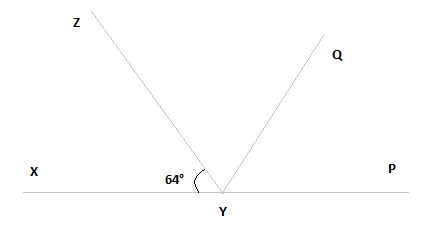Guru

# It is given that XYZ = 64° and XY is produced to point P. Draw a figure from the given information. If ray YQ bisects ZYP, find XYQ and reflex QYP. Q.6

• 0

I want to know the best answer of the question from Lines and Angles chapter of class 9th ncert math. The question from exercise 6.1of math. Give me the easy way for solving this question of 6  It is given that XYZ = 64° and XY is produced to point P. Draw a figure from the given information. If ray YQ bisects ZYP, find XYQ and reflex QYP.

Share

1.Here, XP is a straight line

So, XYZ +ZYP = 180°

Putting the value of XYZ = 64° we get,

64° +ZYP = 180°

∴ ZYP = 116°

From the diagram, we also know that ZYP = ZYQ + QYP

Now, as YQ bisects ZYP,

ZYQ = QYP

Or, ZYP = 2ZYQ

∴ ZYQ = QYP = 58°

Again, XYQ = XYZ + ZYQ

By putting the value of XYZ = 64° and ZYQ = 58° we get.

XYQ = 64°+58°

Or, XYQ = 122°

Now, reflex QYP = 180°+XYQ

We computed that the value of XYQ = 122°.

So,

QYP = 180°+122°

∴ QYP = 302°

• 0